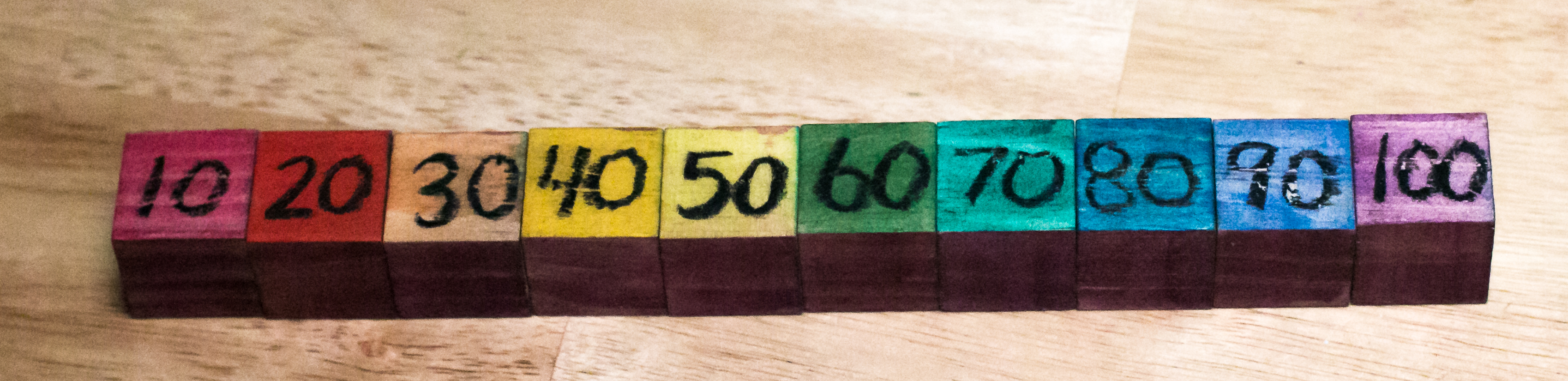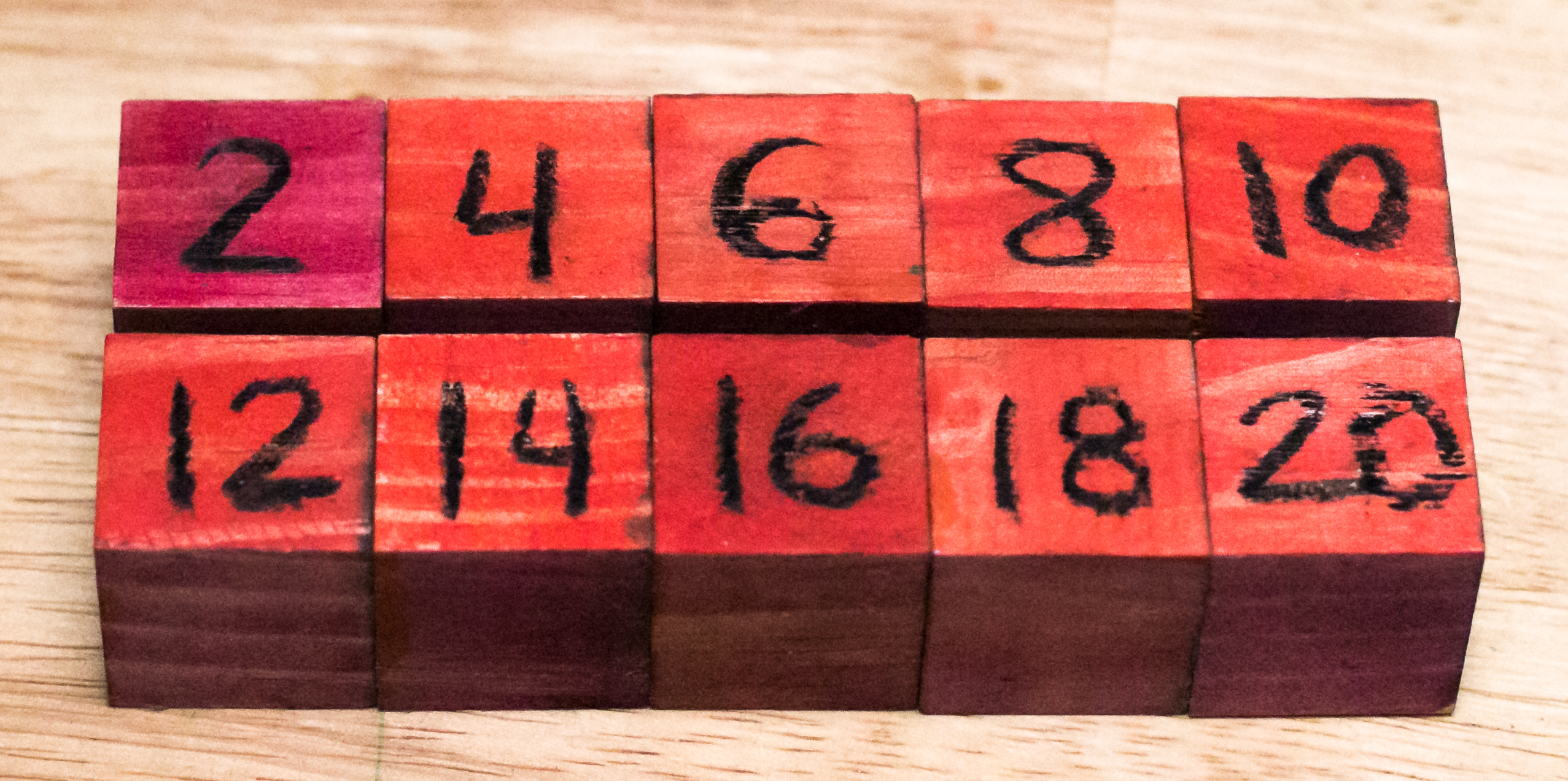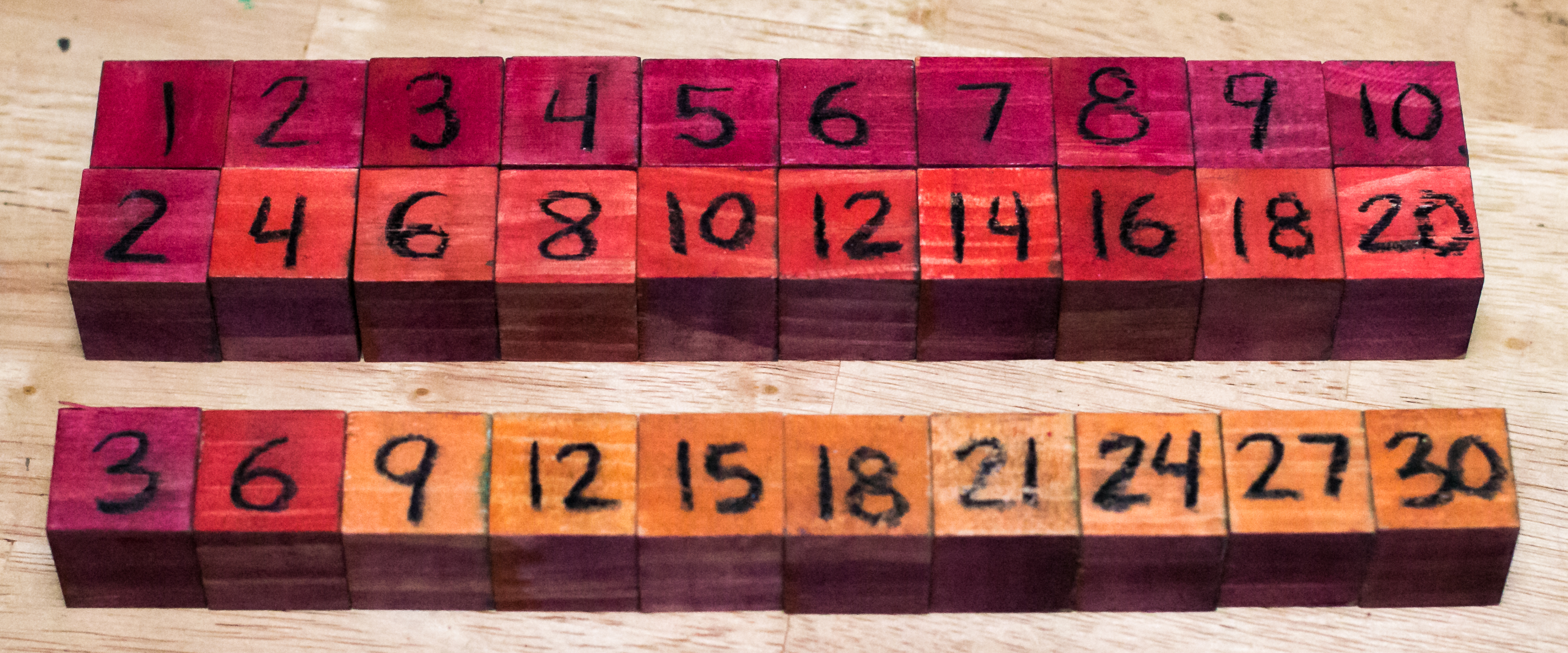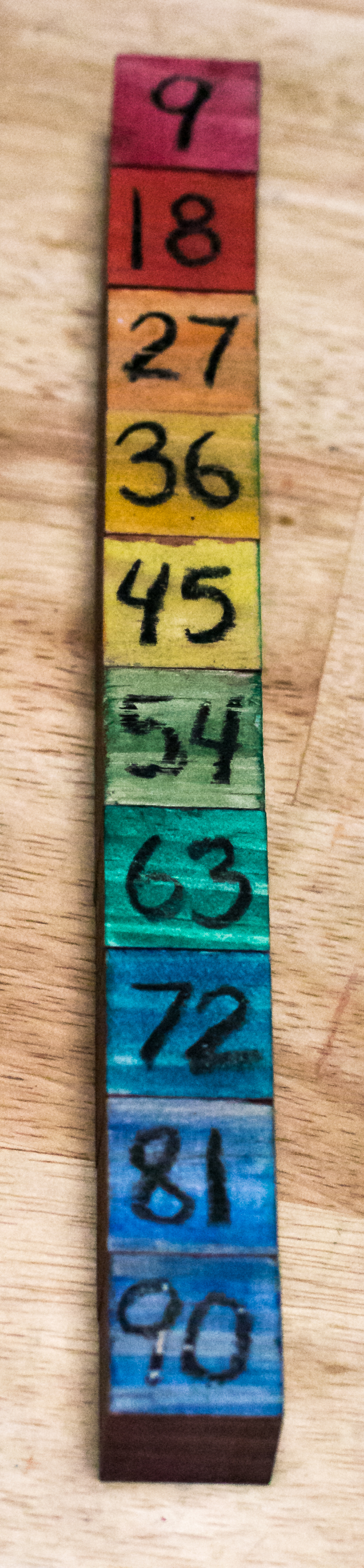# Patterns in the Multiplication Chart

###### *A note about the numbers.  In this piece to try to keep things clear, when I speak of the multiples or a particular number, I am writing the numeric symbol for the number i.e. “9.” If I am not talking about the number but describing an amount, I am spelling it out i.e. “three columns.”  Talking about numbers can get confusing, but I hope that helps.

Introducing the multiplication table can seem daunting for some of us, especially those that remember timed tests and flashcard drills, but it doesn’t have to be that way.  The multiplication chart has some interesting patterns hidden in their depth. I talk about some of them here, but not nearly all of them.

# The Tens

Instead of going in numerical order, it may be easiest to go through the ones that are easiest to memorize and have the simplest patterns and work your way into more complex multiples. The ten’s  pattern is simple, and the rhythm when skip counting  is so easy. The pattern for 10 is pretty easy; you just add a zero to whatever you’re multiplying.# The Fives

The rhythm of 5 is maybe even easier than the rhythm of 10. It definitely has a beat when you skip count to it. One pattern is to just 5 added in between each of the tens. You’ll also notice that the 5’s are half of the tens. So if we’re looking at 10 * 1 is 10, then 5 * 1 is going to be half of that 10, which is 5. If we’re looking at 7 * 10 is 70, then 7 * 5 is going to be half of 70, which is 35.# The Doubles, Double-Doubles, and Double-Double-Doubles

Let’s look at multiples of 2. You’ll notice that the 2’s are just double what the 1’s are. I’m not sure why, but children really seem to enjoy and understand doubles easily, so this makes it a really good tool for introducing and memorizing multiplication chart. When we look at the 4’s, you’ll also notice that the 4’s are double the twos, or you could say double-double the ones. And in that same way 8’s are doubled the 4’s, making them a double-double-double.# More Patterns for the 2’s, 4’s, 6’s & 8’s

If we go back and look at the 2’s and rearrange the multiples of 2 so that the ones digits that are the same fall into the same column, you start to see some interesting patterns 2. If you use the prompts” I wonder” and “I notice,” this is the perfect opportunity to do so. Began by saying,” I notice that in the first column the ones digit is always 2.” Then ask your child what they notice. You can then go on to say,” I wonder if there’s any other pattern in the columns.” I will say that sometimes in order to see some of the patterns, it takes more than just 10 of the multiples. So if you have a 100 chart it might be worthwhile to take that and pull out all the multiples for of 2 to 100, or whatever number you’re working on.  You can also just write the multiples on a sheet of paper, cut them out, and move them around to see what you might find.A similar pattern this type happens for 4’s, 6’s, and 8’s. In the 2’s you’ll also notice that the 2, 6, 8 and 0 repeat themselves in a cycle going across the rows. This also happens in the multiples of 4’s, 6’s and 8’s, but the order of those numbers changes.

# The Three’s and Six’s

Moving on to the 3’s you’ll notice that the 3’s you can get by adding the multiples of 1 in the multiples of 2. This is pretty logical if you think about it, as really you have 2 of something, and you’re just adding 1 more to get the 3. However I would really take the opportunity here to memorize skip counting 3’s and even the 4’s pretty thoroughly. Elementary-age children from about first grade to maybe 5th grade really have an uncanny ability to memorize things. And this memorization skill can be used for things they will need later on, like linking verbs, or the prepositions or the 3’s.Moving onto the 6’s you’ll notice that they are just double the 3’s. So if you can figure out what 3 * 3 is, which is 9, then you will know that 6 * 3 is double nine, 18.If we arrange the 3’s into three columns we begin to notice and other interesting patterns. For instance you’ll notice going down the column the ones digit goes down by one each step.

So in the first column it goes from 3 to 2 to 1 to 0. In the second it starts at 6 and goes down to 5 and 4. And in the third column it begins at 9 and goes 8 to 7. I think it would be really interesting here to ask your children if they think the pattern continue if you added more multiples of 3. This could even be done on paper, and would be a fun way to look for patterns.

# The Nine’s

Now if you look at 9’s you can see the 9’s are triple what 3’s are. So you could say that if 3 * 4 is 12 then 9 * 4 is going to be 3 * 12 which is 36. But I wouldn’t use this particular pattern to learn the multiplication table.As interesting that pattern is, nine is a pretty cool number, and there’s a much better way to find the multiples of 9. If place the 9 multiples into a single column you’ll see that on the ones side going down it decreases by 1 counting backwards 9, 8, 7, 6, 5, 4, 3, 2, 1 and finally making its way to 0. And if you look at the tens place going down the column you’ll know that it goes up by increment of one. Starts at zero and then goes up to 1, 2, 3, 4, 5, 6, 7, 8, and 9. In addition to that you’ll notice if you add the digits 2 any of the multiples of 9, they add up to 9.For example if we look at 9 and 7 which is 63 and we add the 6 and 3 we get 9. So the easy way to find what the multiple of 9 is to take that number we are multiplying by, say 7, and crave it by one.  The tens digit of the product is going to be one less then whatever you’re multiplying 9 by in this case 7.  1 less than 7 is 6, so 6 will be our tens digit. We know that we add those digits together they have to be in 9. Given that information then we knows the ones digit has to be at 3. This makes 9 * 7 to be 63.

# The Seven’s

The 7’s are the only numbers that don’t have a super easy pattern, but we’ve already hit all the other multiples of 7 with the other numbers.  The only number left is 7 * 7, a square number.  One multiplication table is pretty easy to learn.  49

# What do you notice?

This is definitely not an exhaustive list of all the magic this chart contains. What do you notice? Leave a comment below. And if you choose to do an activity that finds these patterns with your children, share them with me by tagging me at @beauty_of_play